Trigonometric Functions and Their Graphs:
The Tangent
(page 2 of 3)

Sections: The sine and cosine, The tangent, The co-functions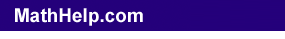The next trig function is the tangent, but that's difficult to show on the unit circle. So let's take a closer look at the sine and cosines graphs, keeping in mind that tan(θ) = sin(θ)/cos(θ).

The tangent will be zero wherever its numerator (the sine) is zero. This happens at 0, π, , , etc, and at –π, –2π, –3π, etc. Let's just consider the region from –π to , for now. So the tangent will be zero (that is, it will cross the x-axis) at –π, 0, π, and .

The tangent will be undefined wherever its denominator (the cosine) is zero. Thinking back to when you learned about graphing rational functions, a zero in the denominator means you'll have a vertical asymptote. So the tangent will have vertical asymptotes wherever the cosine is zero: at –π/2, π/2, and 3π/2. Let's put dots for the zeroes and dashed vertical lines for the asymptotes: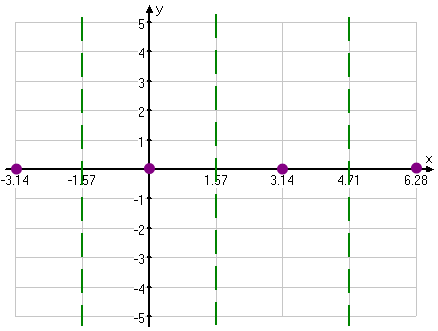Now we can use what we know about sine, cosine, and asymptotes to fill in the rest of the tangent's graph: We know that the graph will never touch or cross the vertical asymptotes; we know that, between a zero and an asymptote, the graph will either be below the axis (and slide down the asymptote to negative infinity) or else be above the axis (and skinny up the asymptote to positive infinity). Between zero and π/2, sine and cosine are both positive. This means that the tangent, being their quotient, is positive, so the graph slides up the asymptote: Copyright © Elizabeth Stapel 2010-2011 All Rights Reserved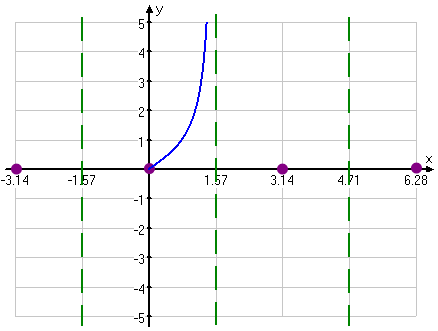Between π/2 and π, sine is positive but cosine is negative. These opposite signs mean that the tangent quotient will be negative, so it will come up the asymptote from below, to meet the x-axis at x = π: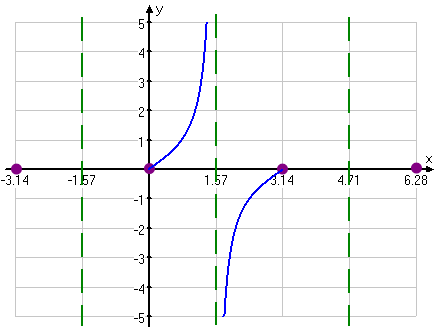Since sine and cosine are periodic, then tangent has to be, as well. A quick check of the signs tells us how to fill in the rest of the graph:

• –π to –π/2: sine is negative and cosine is negative, so tangent is positive
• –π/2 to 0: sine is negative but cosine is positive, so tangent is negative
• π to 3π/2: sine is negative and cosine is negative, so tangent is positive
• 3π/2 to : sine is negative but cosine is positive, so tangent is negative

Now we can complete our graph: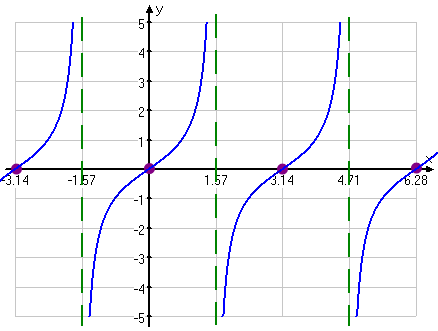The Tangent Graph

As you can see, the tangent has a period of π, with each period separated by a vertical asymptote. The concept of "amplitude" doesn't really apply.

For graphing, draw in the zeroes at x = 0, π, , etc, and dash in the vertical asymptotes midway between each zero. Then draw in the curve. You can plot a few more points if you like, but you don't generally gain much from doing so.

If you prefer memorizing graphs, then memorize the above. But I always had trouble keeping straight anything much past sine and cosine, so I used the reasoning demonstrated above to figure out the tangent (and the other trig) graphs. As long as you know your sines and cosines very well, you'll be able to figure out everything else.

<< Previous  Top  |  1 | 2 | 3  |  Return to Index  Next >>

 Cite this article as: Stapel, Elizabeth. "Trigonometric Functions and Their Graphs: Tangent." Purplemath. Available from     https://www.purplemath.com/modules/triggrph2.htm. Accessed [Date] [Month] 2016

MathHelp.com Courses

Purplemath:

Study Skills Survey